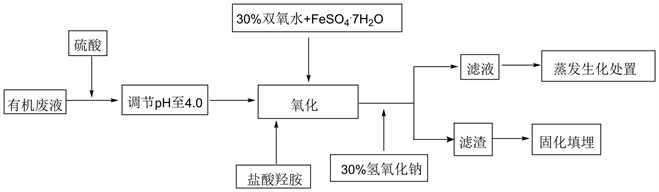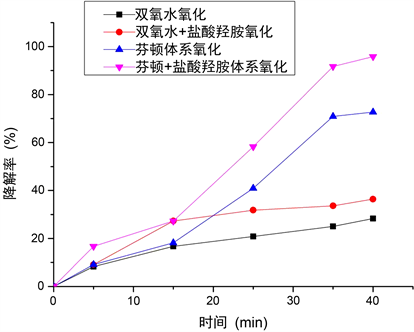#### 期刊菜单

Research on Strengthening the Fenton Oxidation System to Degrade High COD Refractory Waste Liquid

Abstract: Advanced oxidation of organic matter in wastewater is a common treatment method in existing enterprises, which has a good development and application prospect. Fenton oxidation system is the most widely applicable method for the treatment of high COD refractory waste liquid. In this paper, in order to solve the problem of slow reaction efficiency caused by the accumulation of ferric iron in the process of degradation of high COD refractory waste liquid in the traditional Fenton oxidation system, hydroxylamine hydrochloride reductant is introduced into the Fenton oxidation system to strengthen the effect of pretreatment and disposal, so as to efficiently degrade high cod refractory waste liquid.

1. 引言

1) 芬顿药剂的投加量增加。芬顿药剂主要为过氧化氢和亚铁药剂，由于反应中Fe2+向Fe3+的转化过程会抑制芬顿反应产生羟基自由基·OH，造成反应效率降低。因此，为了提高氧化效果需要增加药剂的投加量，尤其是亚铁药剂需要增加，导致芬顿氧化的处理成本大幅度增加。

2) 产生的固体废弃物显著增加。芬顿氧化反应一般是在酸性条件下进行的，在调节pH的过程中会产生大量的铁泥沉淀，造成产生的固体废弃物大幅度提高。

3) 未反应完全残留的过氧化氢会对下一阶段的处理效果造成影响，或者对处理后废液的检测数据作为干扰物质。

2. 概述

2.1. 高COD难降解废液水质指标Table 1. Water quality index of high COD refractory waste liquid

2.2. 工艺流程Figure 1. Process flow chart of high COD refractory waste liquid treatment

3. 实验部分

3.1. 主要实验仪器Table 2. The main equipment and instruments of the experiment

3.2. 主要实验试剂Table 3. The main reagent of the experiment

3.3. 实验方法

3.3.1. 双氧水氧化体系

3.3.2. 双氧水 + 盐酸羟胺氧化体系

3.3.3. 芬顿氧化体系

3.3.4. 芬顿氧化 + 盐酸羟胺体系

4. 结果与讨论

4.1. 不同体系对COD降解率的效果Figure 2. Degradation rate of high COD refractory waste liquid by different systems

$\text{Fe}\left(\text{II}\right)+{\text{H}}_{2}{\text{O}}_{2}\to \text{Fe}\left(\text{III}\right)+{\text{OH}}^{-}+\text{HO}\cdot$ (1)

$\text{Fe}\left(\text{III}\right)+{\text{H}}_{2}{\text{O}}_{2}\to \text{Fe}\left(\text{II}\right)+{\text{HO}}_{2}\cdot +{\text{H}}^{+}$ (2)

$\text{HO}\cdot +{\text{H}}_{2}{\text{O}}_{2}\to {\text{HO}}_{2}\cdot +{\text{H}}_{2}\text{O}$ (3)

$\text{HO}\cdot +\text{Fe}\left(\text{II}\right)\to \text{Fe}\left(\text{III}\right)+{\text{OH}}^{-}$ (4)

$\text{Fe}\left(\text{III}\right)+{\text{HO}}_{2}\cdot \to \text{Fe}\left(\text{II}\right)+{\text{O}}_{2}{\text{H}}^{+}$ (5)

$\text{Fe}\left(\text{II}\right)+{\text{HO}}_{2}\cdot +{\text{H}}^{+}\to \text{Fe}\left(\text{III}\right)+{\text{H}}_{2}\text{O}$ (6)

$\text{Fe}\left(\text{III}\right)+{\text{NH}}_{2}\text{OH}\to \text{Fe}\left(\text{II}\right)+1/2{\text{N}}_{2}+{\text{H}}^{+}+{\text{H}}_{2}\text{O}$ (7)

$2\text{Fe}\left(\text{III}\right)+{\text{NH}}_{2}\text{OH}\to 2\text{Fe}\left(\text{II}\right)+1/2{\text{N}}_{2}\text{O}+2{\text{H}}^{+}+1/2{\text{H}}_{2}\text{O}$ (8)

4.2. 盐酸羟胺投加量对COD去除率的影响Table 8. Effect of hydroxyamide hydrochloride dosage on COD removal rate

4.3. 溶液pH对COD去除率的影响Table 9. Effect of solution pH on COD removal rate

5. 结论

1) 传统芬顿氧化体系中由于三价铁的累积导致芬顿反应效率慢且调碱后有大量污泥沉淀，盐酸羟胺的加入可以缓解三价铁的累积，减少污泥沉淀，实现固体废弃物的减量化。

2) 盐酸羟胺的加入加速了传统芬顿氧化体系中二价铁和羟基自由基的生成，强化了芬顿氧化体系的氧化作用。

3) 盐酸羟胺HO·的淬灭作用较弱，过量加入还原性质的盐酸羟胺不会降低COD的去除率。

4) 溶液pH在4.0左右，芬顿氧化 + 盐酸羟胺体系可以很好降解高COD难降解废液，COD去除效率可达到95.8%以上，即废液中COD含量由120,000 mg/L降至5000 mg/L，为后续处理废液其他工艺提供利好条件，最终实现了高效处理。

5) 同芬顿氧化降解COD相比本文引入了还原剂盐酸羟胺，加速了二价铁的生成，强化了芬顿氧化体系，减少了三价铁的生成，避免了污泥沉淀的增加。且还原剂盐酸羟胺同其他还原剂相比过量的盐酸羟胺不会影响芬顿体系的氧化作用，不会降低COD的去除率。

  张旭, 张思聪, 崔健国. 水资源利用与保护[M]. 北京: 中国建筑工业出版社, 2002.  杨志峰, 冯彦等. 流域水资源可持续利用保障体系——理论与实践[M]. 北京: 化学工业出版社, 环境科学与工程出版中心, 2003.  吴桂芹. 有机废水处理中的高级氧化技术[J]. 林业科技情报, 2010, 42(2): 72-73, 75.  孙晓君, 冯玉杰, 蔡伟民, 周德瑞, 井立强, 王志平. 废水中南降解有机物的高级氧化技术[J]. 化工环保, 2001, 21(5): 264-269.  李花, 沈耀良. 废水高级氧化技术现状与研究进展[J]. 水处理技术, 2011, 37(6): 6-9, 14.  曹磊. 高级氧化技术在水处理中的研究与应用[J]. 污染防治技术, 2008, 21(4): 23-25.  邓洪君. Fenton试剂与其他技术联合在废水处理中的应用[J]. 内蒙古电大学刊, 2007(2): 51-52.  伏广龙, 徐国想, 祝春水, 张猛. 芬顿试剂在废水处理中的应用[J]. 环境科学与管理, 2006, 31(8): 133-135.  陈丽玮, 马军, 李旭春, 等. 一种新型的类芬顿体系对苯甲酸的降解效果研究[J]. 黑龙江大学自然科学学报, 2012, 29(1): 110-116.  蒲丽媛, 郑广宏. Fenton试剂处理含难降解有机物污水的研究进展[J]. 中国科技信息, 2008(19): 26-27, 29.